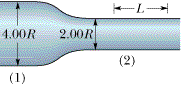# Relating Current Density and Drift Speed

## Homework Statement

The figure shows wire section 1 of diameter 4R and wire section 2 of diameter 2R, connected by a tapered section. The wire is copper and carries a current. Assume that the current is uniformly distributed across any cross-sectional area through the wire's width. The electric potential change V along the length L = 1.95 m shown in section 2 is 13.5 µV. The number of charge carriers per unit volume is 8.49 1028 m-3. What is the drift speed of the conduction electrons in section 1?## Homework Equations

J = nev
v here represents the drift speed
E = pJ
E= V/L
i = nAev
J = i / A

## The Attempt at a Solution

I included a lot of equations on the basis that they have some of the known quantities in them.

I can't really see how to move from knowing what the problem gives me to come up with the drift speed.

I'm given n, the number of charge carriers per unit volume, and the only equations I have that have n in them are J = nev and i = nAev.

So I would need to know J, right? So
J = E / p
and
p = (V/L) / (i/A)
and since i = nAev (and since E = V / L)
I can write
nev = (V/L) / ((V/L) / (nAev)/A)
but then the v cancels out.

So I've gotten no where.